Next: Uncertainty in Be concentration Up: Converting Al and Be Previous: Introduction

# Converting the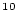Be/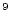Be ratio to theBe concentration in quartz

The accelerator mass spectrometer actually measures the ratio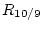of atoms ofBe to atoms ofBe in the target sample. The atoms of both isotopes that actually get to the accelerator can come from a variety of sources: they can be in the actual quartz sample itself, they can be in theBe carrier that is added during chemical processing, or they can be unwanted atoms that arrive sometime during the chemical processing. Thus, the ratiois made up as follows: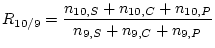(1)

Where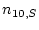is the number of atoms ofBe in the sample;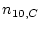is the number of atoms ofBe in the Be carrier (which is supposed to be pureBe, but in reality always contains some amount ofBe);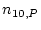is the number of atoms ofBe added accidentally during processing; and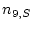,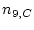and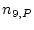are the numbers of atoms ofBe that come from the same three sources.

Note: some AMS labs, Lawrence Livermore in particular, provide two isotope ratios in their results: an uncorrected ratio, and a `blank-corrected' ratio which takes into account one of the process blanks that the user submitted with their sample. The object of this document is to describe how to do the blank correction, sohere refers to the isotope ratio that has not already had the process blank subtracted from it. AMS labs handle this differently in their results, so you should check to determine which value you have.

The quantity we actually want to know is the number of atoms per gram of quartz in the sample, which isn't in this equation yet. Call it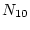, the capital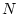denoting a concentration in atoms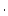g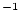rather than a number of atoms.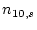, the total number of atoms in the sample, is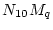, where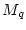is the mass of the quartz sample (g). This makes Equation 1: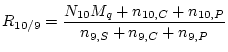(2)

We can simplify this equation in two ways.

First, Be is a rare element in the environment, so (in nearly all cases), the amount ofBe that is in the original sample and occurs from contamination in the lab is very much less than the relatively large amount ofBe that we add in the form of carrier. Basically,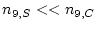and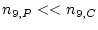, so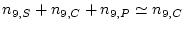.

Second, the standard practice in nearly all laboratories is to use the same amount ofBe carrier for all samples and all process blanks, that is, blanks that are subjected to the entire chemical extraction process in parallel with the samples. Thus, the number of atoms ofBe added with the carrierwill be the same for all samples and process blanks. Also, we have to assume that the number of atoms ofBe added accidentally during processingwill always be the same for all samples, at least within a particular batch of samples processed together. Thus, the quantity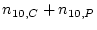will always be the same for samples and process blanks, so we can simply call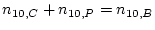, that is, the number of atoms ofBe in a process blank. Section 4 below describes how to decide on a value for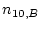.

With these two simplifications we have: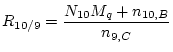(3)

The number of atoms ofBe in the carrieris calculated from the mass of Be added as carrier: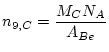(4)

where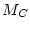is the mass of Be added as carrier (g),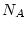is Avogadro's number (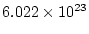atomsmol), and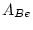is the molar weight of Be (9.012 gmol). For example, most labs add 250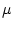g ofBe as carrier, which contains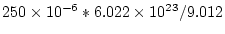=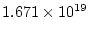atoms ofBe.

This gives: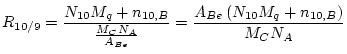(5)

which we can solve to get the formula for the quantity we actually want,: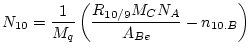(6)Next: Uncertainty in Be concentration Up: Converting Al and Be Previous: Introduction
2006-05-08